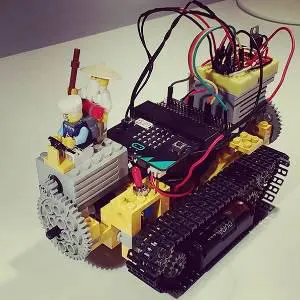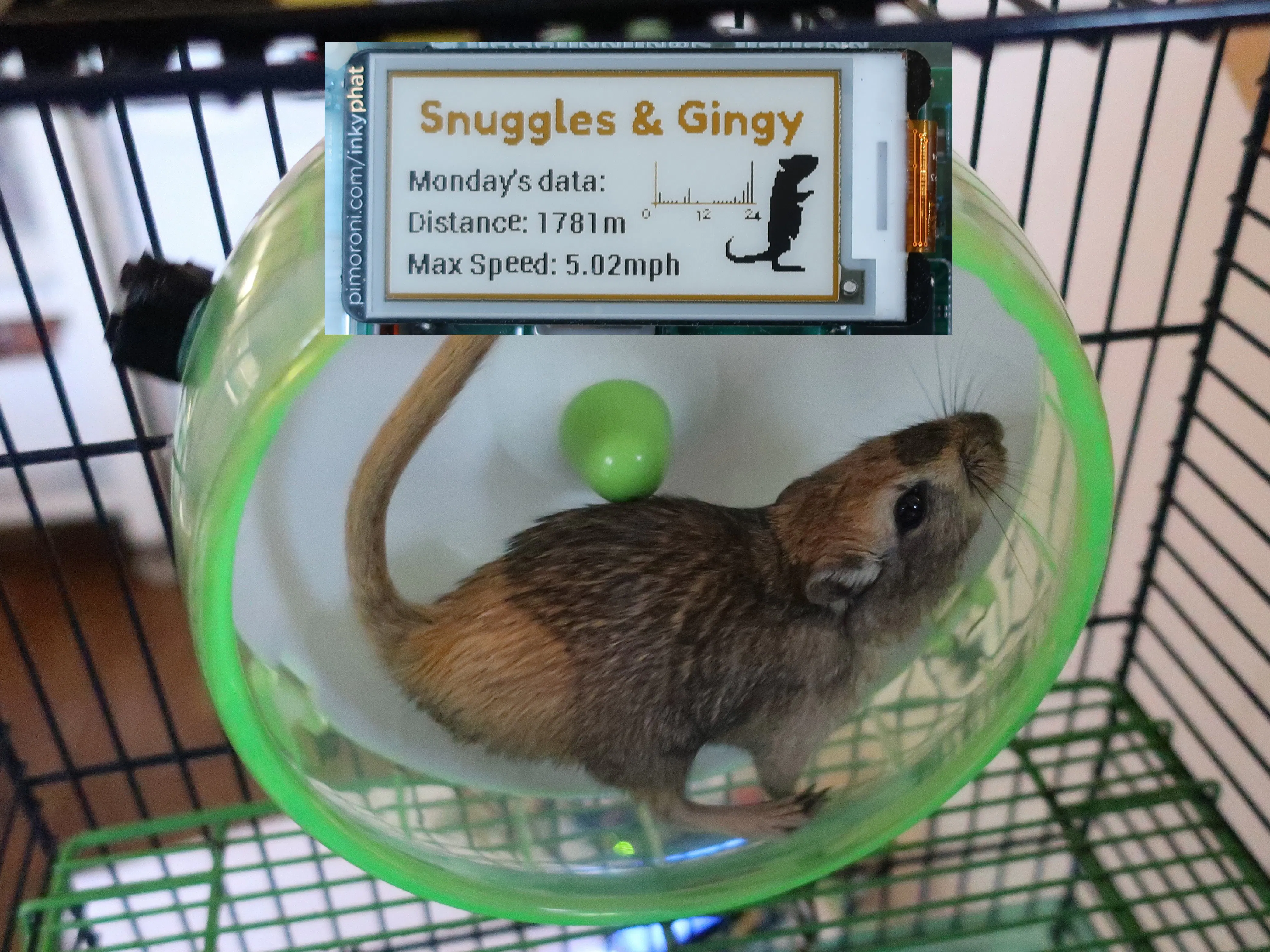Published

# Gerbil Fitbit - Now with Pimoroni ePaper Display!

How far and fast can my gerbils, Snuggles and Gingy, run? IR sensor odometer, with ePaper display of daily stats.

IntermediateShowcase (no instructions)1,750## Things used in this project

### Hardware componentsArduino UNO & Genuino UNO
×1
 IR Break Beam Sensor
×1Pimoroni Inky pHAT
×1Raspberry Pi 3 Model B
×1

### Hand tools and fabrication machinesHot glue gun (generic)

## Code

### Gerbil Exercise Wheel Monitor

Arduino
Measuring the distance and speed of a Gerbil in an exercise wheel.
```// Gerbil exercise wheel monitor
// by Stuart Bryant - 25-Feb-19

// Include the library code:
#include "Wire.h"

// Connect via i2c, default address #0 (A0-A2 not jumpered):

// Define LED pin:
#define LED_PIN 13

// Define IR sensor pin:
#define IR_SENSOR 3

// Define wheel diameter constant [mm]:
#define Diameter 130

// Variable for recording distance:
float Distance = 0;

// Define integer variables:
int Counts = 0; // counts the number of times that the IR beam has been broken
int n = 0; // counter for Header function
int DistInt = 0; // rounded distance

// Define timing variables:
float LastTrigger = 0.0;
float Period = 0.0;
float Speed = 0.0;
float MaxSpeed = 0.0;
float LastSpeed = 0.0;

void setup() {

// Open serial monitor:
Serial.begin(9600);
Serial.println("Gerbil monitor!");
Serial.println("Distance [m] | Speed [mph] | Max Speed [mph]");

// set up the LCD's number of rows and columns:
lcd.begin(16, 2);
// Print a text to LCD:
lcd.clear();
lcd.print("Meters");
lcd.setCursor(0, 1);
lcd.print("Max mph");

// Setup the LED:
pinMode(LED_PIN,OUTPUT);

// Setup the IR sensor input with a pull-up resistor:
pinMode(IR_SENSOR,INPUT_PULLUP);

// Attach interrupt tp IR sensor pin:
attachInterrupt(digitalPinToInterrupt(IR_SENSOR),BeamBreak, FALLING);
}

void BeamBreak() {

Counts++;

// Calculated distance in meters:
Distance = Diameter * 3.14 * Counts / 1000;
DistInt = Distance;
Period = millis() - LastTrigger;
Speed = Diameter / Period * 7.028; // pi x 1000ms x 2.23694 mph/ m/s

if (Speed - LastSpeed < 3) { // filters out false readings

LastSpeed = Speed; // updates max speed
if (Speed > MaxSpeed) {
MaxSpeed = Speed;
}

// Print data to serial monitor:
Serial.print(Distance);
Serial.print("            ");
Serial.print(Speed);
Serial.print("            ");
Serial.println(MaxSpeed);

LastTrigger = millis();
}
}

// Function for formatting the serial monitor output with headers:
if (n > 4) {
Serial.println("Distance [m] | Speed [mph] | Max Speed [mph]");
n = 0;
}
n++;
}

void loop() {

// Illuminate board LED each time IR beam is broken:
digitalWrite(LED_PIN, HIGH);
}
else
{
digitalWrite(LED_PIN, LOW);
}

// Print data to LCD:
lcd.setCursor(9, 0);
lcd.print(DistInt);
lcd.setCursor(9, 1);
lcd.print(MaxSpeed);
}
```

### Gerbil Exercise Wheel Monitor v2 (serial communication with Pi)

Arduino
```// Gerbil exercise wheel monitor
// by Stuart Bryant - 15-Apr-19
// v2 - adds serial communication with Raspberry Pi

// Include the library code:
#include "Wire.h"

// Connect via i2c, default address #0 (A0-A2 not jumpered):

// Define LED pin:
#define LED_PIN 13

// Define IR sensor pin:
#define IR_SENSOR 3

// Define wheel diameter constant [mm]:
#define Diameter 130

// Variable for recording distance:
float Distance = 0;

// Define integer variables:
int Counts = 0; // counts the number of times that the IR beam has been broken
int n = 0; // counter for Header function
int DistInt = 0; // rounded distance
int pi = 0; // serial message from Pi

// Define timing variables:
float LastTrigger = 0.0;
float Period = 0.0;
float Speed = 0.0;
float MaxSpeed = 0.0;
float LastSpeed = 0.0;

void setup() {

// Open serial monitor:
Serial.begin(9600);
//Serial.println("Gerbil monitor!");
//Serial.println("Distance [m] | Speed [mph] | Max Speed [mph]");

// set up the LCD's number of rows and columns:
lcd.begin(16, 2);

displayReset();

// Setup the LED:
pinMode(LED_PIN,OUTPUT);

// Setup the IR sensor input with a pull-up resistor:
pinMode(IR_SENSOR,INPUT_PULLUP);

// Attach interrupt tp IR sensor pin:
attachInterrupt(digitalPinToInterrupt(IR_SENSOR),BeamBreak, FALLING);
}

void displayReset() {
// Print a reading description text to LCD:
lcd.clear();
lcd.print("Meters");
lcd.setCursor(0, 1);
lcd.print("Max mph");
}

void BeamBreak() {

Counts++;

// Calculated distance in meters:
Distance = Diameter * 3.14 * Counts / 1000;
DistInt = Distance;
Period = millis() - LastTrigger;
Speed = Diameter / Period * 7.028; // pi x 1000ms x 2.23694 mph/ m/s

if (Speed - LastSpeed < 3) { // filters out false readings

LastSpeed = Speed; // updates max speed
if (Speed > MaxSpeed) {
MaxSpeed = Speed;
//Serial.println("M"); // disabled in v2
//delay(5);
//Serial.println(MaxSpeed);
}

// Print data to serial monitor:
//Serial.print(Distance); // disabled in v2
//Serial.print("            ");
//Serial.print(Speed);
//Serial.print("            ");
//Serial.println(MaxSpeed);

LastTrigger = millis();
}
}

// Function for formatting the serial monitor output with headers:
if (n > 4) {
Serial.println("Distance [m] | Speed [mph] | Max Speed [mph]");
n = 0;
}
n++;
}

void loop() {

// Illuminate board LED each time IR beam is broken:
digitalWrite(LED_PIN, HIGH);
}
else
{
digitalWrite(LED_PIN, LOW);
}

if(Serial.available()>0){
if(pi == 115){ // if 's' recieved
Serial.println(MaxSpeed);
}
else if(pi == 100) { // if 'd' recieved
Serial.println(Distance);
}
else if(pi == 114) { // if 'r' recieved
Counts = 0;
DistInt = 0;
Distance = 0;
MaxSpeed = 0;
displayReset();
}
else{
Serial.println(pi);
}

}

// Print data to LCD:
lcd.setCursor(9, 0);
lcd.print(DistInt);
lcd.setCursor(9, 1);
lcd.print(MaxSpeed);
}
```

### Inky pHAT Display of Gerbil Data

Python
Code for Raspberry Pi
```# Inky pHAT display of gerbil data
# by Stuart Bryant 15-Apr-19

# Inky pHAT set-up:

from inky import InkyPHAT
import numpy as np
import math
import csv

inky_display = InkyPHAT("yellow")
inky_display.set_border(inky_display.YELLOW)

# Time imports and set-up:
import calendar as c
import time as t
import datetime as dt

weekdays = ['Monday','Tuesday','Wednesday','Thursday','Friday','Saturday','Sunday']

#Set-up serial connection to Arduino:
import serial
ser = serial.Serial('/dev/ttyACM0', 9600) # open serial port to Arduino
ser.flushInput() # remove any data from the serial buffer

# Import Python Image Library:
from PIL import Image, ImageFont, ImageDraw

# Create a new canvas to draw on:
img = Image.open("/home/pi/GerbilProject/G6.png")
draw = ImageDraw.Draw(img)

# Font definitions:
from font_fredoka_one import FredokaOne
font = ImageFont.truetype(FredokaOne, 22)
font2 = ImageFont.truetype("Piboto-Bold.ttf", 14)
font3 = ImageFont.truetype("Piboto-Bold.ttf", 7)

# Create array for storing hourly distance:
dist_array = np.full(24,1)

# Create array for storing daily distance:
week_array = np.full(7,0)

# Import previous 24 hours of data:
with open('24hrData.csv', 'rt') as f:
for n in range (0, 24):
dist_array[n] = line[n]
f.close()

# Import previous daily distances:
with open('WeekData.csv', 'rt') as f:
for n in range (0, 7):
week_array[n] = line[n]
f.close()

m = np.amax(dist_array) # maxiumum hourly distance
maxSpeed = 0 # maximum speed variable

def screenUpdate():
# Create a new canvas to draw on
img = Image.open("/home/pi/GerbilProject/G6.png")
draw = ImageDraw.Draw(img)

# Title text:
title = "Snuggles & Gingy"
w, h = font.getsize(title)
x = (inky_display.WIDTH / 2) - (w / 2)
y = 7
draw.text((x,y), title, inky_display.YELLOW, font)

# Data lines:
textDay = yesterday() + "'s data:"
draw.text((8,40), textDay, inky_display.BLACK, font2)

textDistance ="Distance: " + str(np.sum(dist_array)) + "m"
draw.text((8,60), textDistance, inky_display.BLACK, font2)

textSpeed ="Max Speed: " + str(maxSpeed) + "mph"
draw.text((8,80), textSpeed, inky_display.BLACK, font2)

m = np.amax(dist_array)
# Draw histogram data:
for n in range (0, 24):
draw.line((129+n*2,60, 129+n*2,60-dist_array[n]/m*20), fill=1)

# Histogram axes:
draw.line((127,40, 127,62), fill=2)
draw.line((125,60, 175,60), fill=2)

# x-axis labels:
draw.text((121,62), "0", inky_display.BLACK, font3)
draw.text((146,62), "12", inky_display.BLACK, font3)
draw.text((170,62), "24", inky_display.BLACK, font3)

# Set image and display:
inky_display.set_image(img)
inky_display.show()

def yesterday():
yesterday = weekdays[c.weekday(t.gmtime().tm_year,t.gmtime().tm_mon,t.gmtime().tm_mday)-1]
return yesterday

def ArduinoSerial(input):
ser.write(bytes(input.encode('ascii')))
t.sleep(0.1) # wait for Arduino to respond
if(ser.inWaiting() >0):
output = ""
l = len(line)
l = l - 2
for char in line:
output = output + chr(char)
outputFloat = float(output[:l])
return outputFloat

screenUpdate()

step = 3600

s = t.time()
s = math.ceil(s/step)*step
interval = step # one hour interval
lastDistance = 0

while 1:
if (t.time() > s):
distance = ArduinoSerial('d')
maxSpeed = ArduinoSerial('s')

lastDistance = distance

s = s + interval

print("###################################")
print("Time: "+str(t.gmtime().tm_hour)+":"+str(t.gmtime().tm_min)+":"+str(t.gmtime().tm_sec))
print("Interval distance =",deltaDistance, "Total distance:", distance)
print("Max speed today: "+str(maxSpeed))
print("Distance array (last 24 hours):")
print(dist_array)

# Save last 24 hours of data:
with open('24hrData.csv', 'wt') as f:
csv_writer = csv.writer(f, delimiter=',', quoting=csv.QUOTE_NONNUMERIC)
csv_writer.writerow(dist_array)
f.close()

# Midnight posting of th days stats:
if (t.gmtime().tm_hour == 0):

week_array[c.weekday(t.gmtime().tm_year,t.gmtime().tm_mon,t.gmtime().tm_mday)-1] = np.sum(dist_array)

# Save daily data:
with open('WeekData.csv', 'wt') as f:
csv_writer = csv.writer(f, delimiter=',', quoting=csv.QUOTE_NONNUMERIC)
csv_writer.writerow(week_array)
f.close()

screenUpdate()
ser.write(b'r') # to reset Arduino counter
t.sleep(0.1)
ser.flushInput()
lastDistance = 0
```

## Credits

### hackminster

0 projects • 1 follower